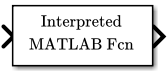# Interpreted MATLAB Function

Apply MATLAB function or expression to input

• Library:

•## Description

The Interpreted MATLAB Function block applies the specified MATLAB® function or expression to the input. The output of the function must match the output dimensions of the block.

Some valid expressions for this block are:

```sin atan2(u(1), u(2)) u(1)^u(2) ```

### Note

This block is slow because it calls the MATLAB parser during each integration step. Consider using built-in blocks (such as the Math Function block) instead. Alternatively, you can write the function as a MATLAB S-function or MEX-file S-function, then access it using the S-Function block.

## Ports

### Input

expand all

The Interpreted MATLAB Function block accepts one real or complex input of type `double` and generates real or complex output of type `double`, depending on the setting of the Output signal type parameter.

Data Types: `double`

### Output

expand all

The Interpreted MATLAB Function block accepts one real or complex input of type `double` and generates real or complex output of type `double`, depending on the setting of the Output signal type parameter.

Data Types: `double`

## Parameters

expand all

Specify the function or expression. If you specify a function only, it is not necessary to include the input argument in parentheses.

Specify the dimensions of the block output signal, for example, `2` for a two-element vector. The output dimensions must match the dimensions of the value returned by the function or expression in the MATLAB function field.

Specify `-1` to inherit the dimensions from the output of the specified function or expression. To determine the output dimensions, Simulink runs the function or expression once before simulation starts.

### Note

If you specify `-1` for this parameter and your function has persistent variables, then the variables might update before the simulation starts. If you need to use persistent variables, consider setting this parameter to a value other than `-1`.

Specify the output signal type of the block as `real`, `complex`, or `auto`. A value of `auto` sets the output type to be the same as the type of the input signal.

Select this check box to output a 2-D array as a 1-D array containing the 2-D array's elements in column-major order.

### Note

This parameter is not visible in the block dialog box unless it is explicitly set to a value other than `-1`. To learn more, see Blocks for Which Sample Time Is Not Recommended.

## Block Characteristics

 Data Types `double` Direct Feedthrough `yes` Multidimensional Signals `no` Variable-Size Signals `no` Zero-Crossing Detection `no`